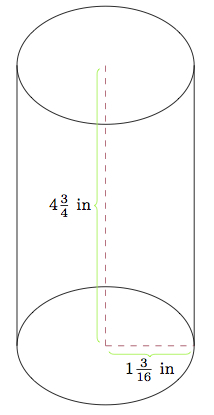# How thick is a soda can? Variation I

Alignments to Content Standards: G-MG.A.1 G-MG.A.2

A cylindrical soda can is made of aluminum. It is approximately $4 \frac{3}{4}$ inches high and the top and bottom have a radius of approximately $1 \frac{3}{16}$ inches:1. Find the approximate surface area of the soda can. What assumptions do you use in your estimate?
2. The density of aluminum is approximately $2.70$ grams per cubic centimeter. If the mass of the soda can is approximately $15$ grams, how many cubic centimeters of aluminum does it contain?
3. Using the answers to (a) and (b) estimate how thick the aluminum can is.

## IM Commentary

As written, this problem gives students all of the information they need to estimate the thickness of a soda can. Alternatively, they can be given less information and requested to develop a strategy for estimating how thick the can is: this is the approach taken in ''How thick is a soda can II''. An intermediate more hands-on possibility is also available: namely, students could be given a soda can and asked to find its approximate surface area. They could also be asked to find its approximate mass. This will of course require relatively accurate balances and tape measures and is perhaps more suitable for a science class but if these are available it is a good way to involve the students in more active learning.

Here we chose to present the density of aluminum in grams per cubic centimeter because this is the way it is normally listed in a textbook or online resource. If the teacher prefers to give the measurements of the can in centimeters then students will not need to make a conversion in part (c) of this problem but this unit conversion is also good and important work for the students.

This task is suitable for instruction or for assessment although students will require access to a calculator. Its main goal is to provide a familiar context and a straightforward question which require a variety of tools to solve: modeling a situation with geometry, paying close attention to units, and converting units.

It is worth noting that the shape of most soda cans is only approximately cylindrical as they are usually tapered at the top and bottom. There are also issues regarding how accurate the different measurements are and how this should be recorded. This is an issue for measuring the circumference and height of the can as well as the mass of the can. These inaccuracies are then perpetuated when the arithmetic is performed to estimate the thickness. In the end, however, the most vital hypothesis influencing the level of accuracy is probably the assumption that the thickness of the can is uniform: this is not true because the lips on the top and the bottom are thicker and the ''sides'' of the can are actually the thinnest part. Some more detailed information about the actual specifications of an aluminum can can be found here:

Specifications of an Aluminum Can

## Solution

1. The surface of the can is made up of the top and bottom together with the cylindrical part of the can. In practice, the top and bottom are not flat but are well approximated by circles. According to the given information, the radius of these circles is about $1 \frac{3}{16}$ inches. So the area is about $\pi \times \left(1 \frac{3}{16}\right)^2$ square inches or about $4.4$ square inches. If the cylindrical part of the can were made flat, it would be a rectangle with dimensions $4 \frac{3}{4}$ inches by $\pi \times 2 \times 1 \frac{3}{16}$ inches or about $35.4$ square inches. So the total surface area of the can is about $44.2$ square inches.
2. If there are about $15$ grams of aluminum in the soda can and the density of aluminum is about $2.70$ grams per cubic centimeters then there are about $$\frac{15 \mbox{ grams}}{2.70 \mbox{ grams per cubic centimeter}} \approx 5.6 \mbox{ cubic centimeters}$$ of aluminum in the soda can.
3. Since the amount of aluminum is given in cubic centimeters and the area of the soda can in square inches we need to make a conversion. There are about 2.54 centimeters per inch and so there are about $(2.54)^2 \approx 6.5$ square centimeters per square inch. So $$44.2 \mbox{ square inches} \approx (44.2 \times 6.5) \mbox{ square centimeters}=287.3\text{ square centimeters}.$$ To find the approximate thickness of the can we know, assuming that the thickness of the can is close to uniform, that $${\rm thickness} \times 287.3 \mbox{ cm}^2 \approx 5.6 \mbox{ cm}^3.$$ This means that the approximate thickness of the can is about $0.02$ centimeters or $0.2$ millimeters.